# RS Aggarwal Class 8 Solutions Chapter 17 Construction of Quadrilaterals Ex 17A

## RS Aggarwal Class 8 Solutions Chapter 17 Construction of Quadrilaterals Ex 17A

These Solutions are part of RS Aggarwal Solutions Class 8. Here we have given RS Aggarwal Solutions Class 8 Chapter 17 Construction of Quadrilaterals Ex 17A.

Other Exercises

Question 1.
Solution:
Steps of Construction :
(i) Draw a line segment AB = 4.2 cm.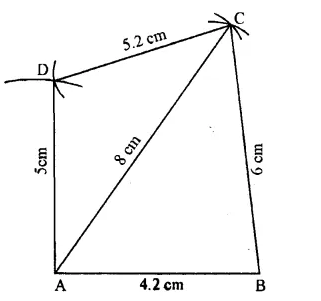(ii) With centre A and radius 8 cm and with centre B and radius 6 cm., draw arcs intersecting each other at C.
(iii) Join AC and BC.
(iv) Again with centre A and radius 5 cm. and with centre C, radius 5 2 cm. draw arcs intersecting each other at D.

Question 2.
Solution:
Steps of Construction :
(i) Draw a line segment PQ = 5.4 cm.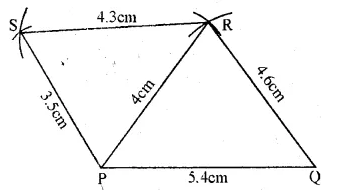(ii) With Centre P and radius 4 cm. and with centre Q and radius 4.6 cm., draw arcs intersecting each other at R.
(iii) Join PR and QR.
(iv) Again with centre P and radius 3.5 cm. and with centre R and radius 4.3 cm. draw arcs intersecting each other at S.
(v) Join PS and RS. PQRS is the required quadrilateral.

Question 3.
Solution:
Steps of Construction :
(i) Draw a line segment AB = 3.5 cm.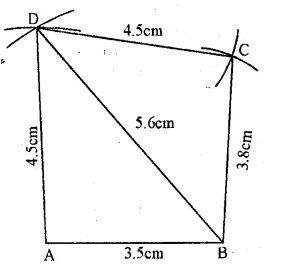(ii) With centre A and radius 4.5 cm. and with centre B and radius 5.6 cm. draw arcs intersecting each other at D.
(iv) With centre B and radius 3.8 cm. and with centre D and radius 4.5 cm., draw arcs intersecting each other at C.
(v) Join BC and DC. ABCD is the required quadrilateral.

Question 4.
Solution:
Steps of Construction :
(i) Draw a line segment AB = 3.6 cm.
(ii) With centre A and radius 4.6 cm. and with centre B and radius 3.3 cm. draw arcs intersecting each other at C.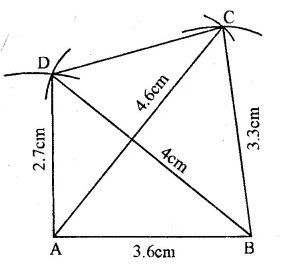(iii) Join AC and BC.
(iv) Again with centre A and radius 2.7 cm. and centre B and radius 4 cm., draw arcs intersecting each other at D.

Question 5.
Solution:
Steps of Construction :
(i) Draw a line segment RS = 5 cm.
(ii) With centre R and S, radius 6 cm. each, draw arcs intersecting each other at R
(iii) Join PR and PS.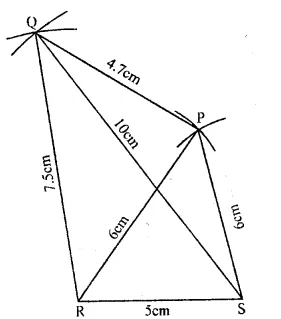(iv) With centre R and radius 7.5 cm. and with centre S and radius 10 cm, draw arcs intersecting each other at Q.
(v) Join RQ, SQ and PQ. PQRS is the required quadrilateral. Measuring the fourth sides PQ, it is 4.7 cm. (approx.)

Question 6.
Solution:
Steps of Construction :
(i) Draw a line segment AB = 3.4 cm.(ii) With centre A and radius 5.7 cm. and with centre B and radius 4 cm., draw arcs intersecting each other at D.
(iv) Again with centre A and radius 8 cm and with centre D and radius 3 cm., draw arcs intersecting each other at C.
(v) Join AC, BC and DC. ABCD is the required quadrilateral

Question 7.
Solution:
Steps of Construction :
(i) Draw a line segment AB = 3.5 cm.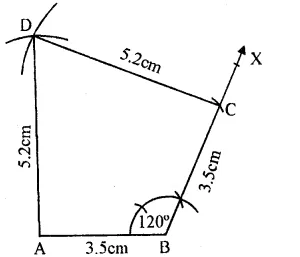(ii) At B, draw a ray BX making an angle of 120° using protractor and cut off BC = 3.5 cm
(iii) With centres A and C and radius 5.2 cm, draw arcs intersecting each other at D.

Question 8.
Solution:
Steps of Construction :
(i) Draw a line AB = 2.9 cm.
(ii) At A, draw a ray AX making an angle of 70° with AB. Using protractor and cut off AD = 3.4 cm.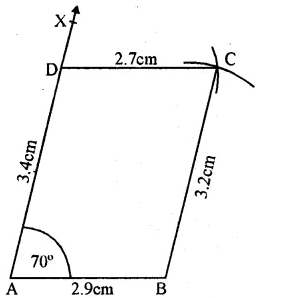(iii) With centre B and radius 3.2 cm and with centre D and radius 2.7 cm., draw arcs intersecting each other at C.
(iv) Join BC and DC. ABCD is the required quadrilateral.

Question 9.
Solution:
Steps of Construction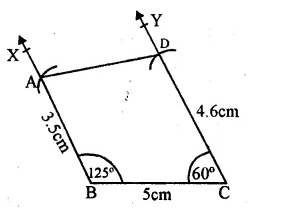(i) Draw a line segment BC = 5 cm.
(ii) At B, draw a ray BX making an angle of 125° and cut off BA = 3.5 cm.
(iii) At C, draw a ray CY making an angle of 60° and cut off CD = 4.6 cm

Question 10.
Solution:
Steps of Construction :
(i) Draw a line segment QR = 5.6 cm.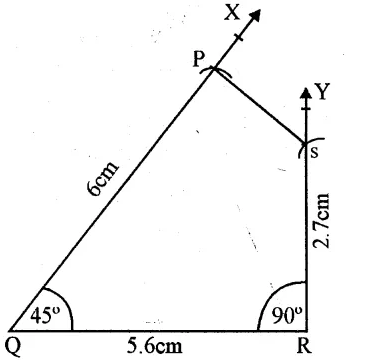(ii) At Q, draw a ray QX making an angle of 45° and cut off QP = 6 cm.
(iii) At R, draw a ray RY making an angle of 90° and cut off RS = 2.7 cm.
(iv) Join SP PQRS is the required quadrilateral.

Question 11.
Solution:
Steps of Construction :
∠A = 50°, ∠B = 105° and ∠D = 80°
and ∠A + ∠B + ∠C + ∠D = 360°
=> 50° + 105° + ∠C + 80° = 360°
=> ∠C + 235° = 360°
=> ∠C = 360° – 235°
=> ∠C = 125°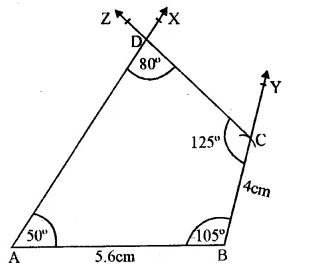(i) Draw a line segment AB = 5.6 cm.
(ii) At B, draw a ray BY making an angle of 105° and cut off BC = 4 cm.
(iii) At C, draw a ray CZ making an. angle of 125° and at A, a ray AX making an angle of 50° intersecting each other at D.
then ∠D = 80°

Question 12.
Solution:
∠P + ∠Q + ∠R + ∠S = 360°
100° + ∠Q + 100° + 75° = 360°
=> ∠Q + 275° = 360°
=> ∠Q = 360° – 275°
∠Q = 85°
Steps of Construction :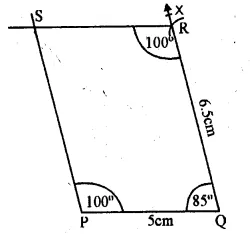(i) Draw a line segment PQ = 5 cm.
(it) At Q, draw a ray QX making an angle of 85° and cut off QR = 6.5 cm.
(iii) At R, draw a ray making an angle of 100° and at P, another ray making an angle of 100° which intersect each other at S. then ∠S = 75°

Question 13.
Solution:
Steps of Construction :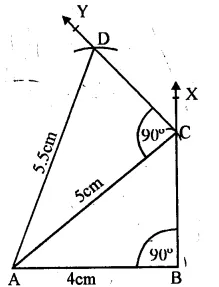(i) Draw a line segment AB = 4 cm.
(ii) At B, draw a ray BX making an angle of 90°.
(iii) From A, draw an arc of 5 cm. radius intersecting BX at C.
(iv) Join AC.
(v) At C, draw a ray CY making an angle of 90°.
(vi) From A, draw an arc of radius 5.5 cm. which intersects CY at D.